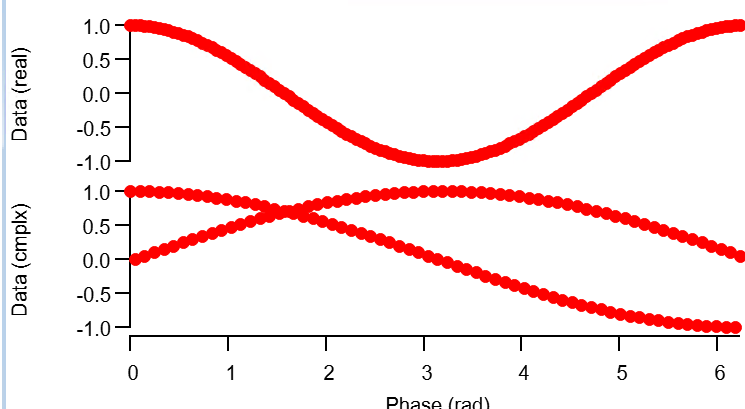# Plotting of complex wave vs real x-value waveI find the plotting of complex wave vs real x-value wave to be nonintuitive.

If the trace is in a non-complex (e.g. real only) mode, the entire wave is displayed.

If the trace is in complex (real & imaginary) mode, only the first half of the wave is displayed.

This is especially confusing to the unaware user as the default setting is to plot complex waves in complex (real & imaginary) trace mode.

While it becomes obviously a bit murky for irregularly spaced (or even non-monotonic) x-value waves, I would suggest that for the complex trace mode, the real value is plotted against the corresponding x-wave value and the imaginary value is e.g. plotted with a small fixed x offset relative to the real value.

Example:

function plotComplex()
make/o/n=128 datax  = 2*pi*p/128
make/o/c/n=128 data = p2rect(cmplx(1,datax[p]))

display data vs datax
appendToGraph/l=l2 data vs datax
ModifyGraph axisEnab(left)={0,0.45},axisEnab(l2)={0.55,1},freePos(l2)=0
ModifyGraph cmplxMode(data)=0, cmplxMode(data#1)=1
ModifyGraph mode=3,marker=19
Label left "Data (cmplx)"
Label l2 "Data (real)"
Label bottom "Phase (rad)"
ModifyGraph lblPos(l2)=100
end